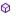Press on the image to return to the main documentation page.

# MFParser

MF_MathParser

### MF_MathParser

This is an 'Activity Object', it cannot be declared under Sub Process_Globals.

#### Events:

Calc(name As String, values() As Double) As Double

#### Members:Copyright As StringCreateUserFunction (funcName As String, paramCount As Int, eventName As String) As BooleanParse (formula As String) As DoubleVariableClearVariableExist (name As String) As BooleanVariableGet (name As String) As DoubleVariableRemove (name As String)VariableSet (name As String, value As Double)Version As String

#### Members description:

#####CopyrightAsString

Example:
Sub Globals
Dim MF_MathParser As MF_MathParser
End Sub

Sub Activity_Create(FirstTime As Boolean)
End Sub
#####CreateUserFunction (funcNameAsString, paramCountAsInt, eventNameAsString) AsBoolean
Create user defined function. The function raises an event on call.
The function name will be forwarded to the event. Therefore you can create a sub for each function or one sub and then select by function name in event.

Example:
Sub Globals
Dim MF_MathParser As MF_MathParser
End Sub

Sub Activity_Create(FirstTime As Boolean)
MF_MathParser.CreateUserFunction("test", 2, "TestEvent")
Dim result As Double
result = MF_MathParser.Parse("test(5,7)")
End Sub

Sub TestEvent_Calc(name As String, values() As Double) As Double
Dim result As Double = 0.0
For Each v In Values
result = result + v
Next
return result
End Sub
#####Parse (formulaAsString) AsDouble
Parses the given formula and returns the result.
Multiple formulas can be used, separated with ";".
If a formula begins with "variable=", this variable will be set with the result.
Additionally a variable "result" with the result value of the last formula will be set.

Example:
Sub Globals
Dim MF_MathParser As MF_MathParser
End Sub

Sub Activity_Create(FirstTime As Boolean)
Dim result As Double
result = MF_MathParser.Parse("x=7;12*(x-2)/5")
End Sub
#####VariableClear
Remove all variables.

Example:
Sub Globals
Dim MF_MathParser As MF_MathParser
End Sub

Sub Activity_Create(FirstTime As Boolean)
MF_MathParser.VariableClear
End Sub
#####VariableExist (nameAsString) AsBoolean
Check if variable exist.

Example:
Sub Globals
Dim MF_MathParser As MF_MathParser
End Sub

Sub Activity_Create(FirstTime As Boolean)
Dim result As Boolean
result = MF_MathParser.VariableExist("x")
End Sub
#####VariableGet (nameAsString) AsDouble
Get value from variable.

Example:
Sub Globals
Dim MF_MathParser As MF_MathParser
End Sub

Sub Activity_Create(FirstTime As Boolean)
Dim result As Double
result = MF_MathParser.VariableGet("x")
End Sub
#####VariableRemove (nameAsString)
Remove variable.

Example:
Sub Globals
Dim MF_MathParser As MF_MathParser
End Sub

Sub Activity_Create(FirstTime As Boolean)
MF_MathParser.VariableRemove("x")
End Sub
#####VariableSet (nameAsString, valueAsDouble)
Set variable with value.

Example:
Sub Globals
Dim MF_MathParser As MF_MathParser
End Sub

Sub Activity_Create(FirstTime As Boolean)
Dim result As Double
MF_MathParser.VariableSet("x", 12)
result = MF_MathParser.Parse("x*(7-2)/5")
End Sub
#####VersionAsString
Returns the MFParser version.

Example:
Sub Globals
Dim MF_MathParser As MF_MathParser
End Sub

Sub Activity_Create(FirstTime As Boolean)
Dim version As String
version = MF_MathParser.Version
End Sub

Top